The compound and continuous interest formulas for seeing money growth over time are very useful, but one resource that is much harder to find is a tool that lets you periodically input money into the total amount as it grows. While it's neat to see how a fixed amount of money grows without touching it, the much more practical application is figuring out the growth over time as you're adding more funds into an account. I will not only provide a calculator for such a need, but also explain the math behind what it will take to calculate something like this.

If you are not interested, you can just go straight to the calculator below to determine how interest and repeated deposits affect your total balance over time.

## Calculator

Initial Amount

The number of times to apply interest over a year (use 365 for daily interest, or 12 for monthly interest)

Deposit amount after every application of interest
(If the previous input was 365 for daily, then you should put the amount of money you deposit daily)
(If the previous input was 12 for monthly, then you should put the amount of money you deposit monthly)

Interest rate (from 0 to 1, as a decimal)

The number of years to wait for growth

NOTE: Due to how the formula works, there is an initial deposit applied at the 0th year before interest is applied!

Your total sum will be NaN

## Explanation

Before the dive into the more complicated formula, it's worth quickly reviewing what the original compound formula is and how it works.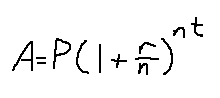P is the initial amount of money to work with

r is the interest rate in decimal format. So, 5% interest translates to r = .05

n is the number of divisions to make for compounding in a year. So, to apply interest once a year, set n = 1. To apply interest once a month, set n = 12.

t is the number of years to apply the interest

What is happening is that every application of the interest means multiplying the current value by that value inside the parentheses. Here's an example of daily interest being applied for the year. Starting with the principal value P, every day means multiplying it by some value.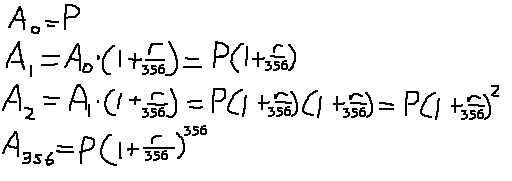The exponent is really representing the number of compound interests to apply, and the unit of that exponent depends on the value under the r. Since we used a daily application of compound interest, the denominator value is 356, and so the exponent is the number of days of growth.

Simple enough. But what if we want to add a fixed amount of money after the application of interest? For example, the interest is applied monthly, and for every month a hundred dollars is added. We will use a similar strategy as the previous image, where we go through every step of interest application, and see what happens. So, in addition to applying the interests, we will add a fixed amount of money to the total balance at the end. This will be represented as P.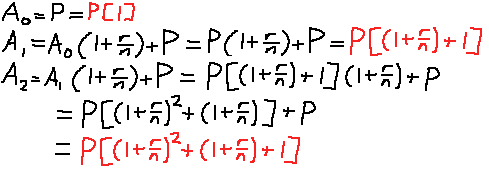Do you see the pattern behind the red colored equations after every application? Every term is some power of (1 + r/n)^K. Yes, even the term of 1. Any term to the power of 0 is 1, after all (yes, I know zero is an exception).

It's fairly easy to generalize this for some K number of interest iterations, if we use summation notation: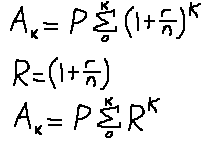To make things easier to write, R is assigned to that rate term in parentheses. At this point, we must rely on the geometric sum formula in order to remove that ugly summation. See below for a reminder of the formula: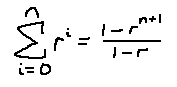After applying that formula, this is our final result: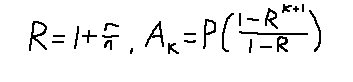To reiterate, P is the amount of money put in after every interest application, k is the number of interests to apply, r is the interest rate, and n is the number of interest applications per year.

Example time: find the total amount of money gained when putting in \$20 a day, with 5% interest, and doing it over 40 years with daily accrued interest.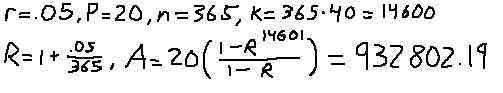For comparison, just putting in \$20 a day for 40 years without any interest would give you \$292,000. That's about a 3.19 factor between the two values.

Here's what the total amount is after applying interest under the same conditions, but monthly instead of daily. Since monthly is "less continuous" than daily, then the total amount should be less than the daily interest. Since the interest is applied monthly, the daily amount of cash deposits must be converted to monthly cash deposits. For approximation, we'll just multiply the \$20 value by 30 days.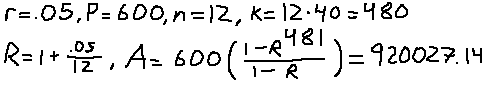This is all very nice, but what if we wanted to calculate this growth over time with a principal amount, just like with the original formula? This formula assumes that you start with a zero balance. Well, if we try and go through the process of finding a formula, we will find that it is surprisingly easy to calculate what value the principle amount adds to. The cool part is that the fixed amount and the variable amount inputted into the balance can be calcuated separately! P0 will be the principle value, while P will remain to be the amount added to the balance for every interest application.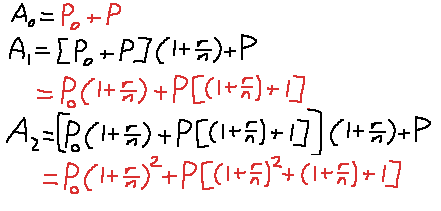Notice how the red term on the left is the new principle value term, while the term on the right is exactly the same value as the one in the previous formula that we already solved. Putting it all together, this is what we get: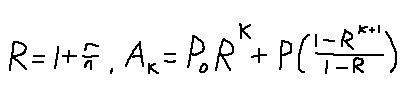As a final test of knowledge, see if you come up with the same values from the following scenario. If you were to invest \$20 a day for the next 40 years, with 5% and a daily interest compound, and 0 initial investment, you should end up with \$932,802.19. But, if you had a \$146,000 initial investment, but contributed only \$10 over the next 40 years (in either scenario you contribute the same amount of money), with 5% interest, and a daily interest compound, you end up with \$1,545,055.53.